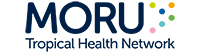Modelling of Infectious Disease CEntre Imperfect Gold Standard Models Subscribe : MODEL106 : 4-tests in 1-population Model (Advance Interface)Please regsiter for subscribe before submit parameter and run the model
If already subscribe please enter user name and password on top of the page
Data input
 Test A Test B Test C Test D Frequency observed Positive Positive Positive Positive Positive Positive Positive Negative Positive Positive Negative Positive Positive Positive Negative Negative Positive Negative Positive Positive Positive Negative Positive Negative Positive Negative Negative Positive Positive Negative Negative Negative Negative Positive Positive Positive Negative Positive Positive Negative Negative Positive Negative Positive Negative Positive Negative Negative Negative Negative Positive Positive Negative Negative Positive Negative Negative Negative Negative Positive Negative Negative Negative Negative
 Correlation between diagnostic tests (Using example value)
 Prior distributions (Using example value) Note! Model default value of specificity of Test A is fixed to 100%. The dataset is taken from a study of diagnostic tests for melioidosis. Test A is culture, and a positive culture of B. pseudomallei is a definite indication of melioidosis infection. Please refer to Limmathurotsakul D, et al. PLoS One 2010;5:e12485 for more details.
 Initial values (Using example value) Note! The prevalance is set to a fixed value in the above section; therefore, its initial will not be taken into account during Bayesian LCM analysis. The sensitivity of test A is set to a fixed value in the above section; therefore, its initial will not be taken into account during Bayesian LCM analysis. The sensitivity of test B is set to a fixed value in the above section; therefore, its initial will not be taken into account during Bayesian LCM analysis. The sensitivity of test C is set to a fixed value in the above section; therefore, its initial will not be taken into account during Bayesian LCM analysis. The sensitivity of test D is set to a fixed value in the above section; therefore, its initial will not be taken into account during Bayesian LCM analysis. The specificity of test A is set to a fixed value in the above section; therefore, its initial will not be taken into account during Bayesian LCM analysis. The specificity of test B is set to a fixed value in the above section; therefore, its initial will not be taken into account during Bayesian LCM analysis. The specificity of test C is set to a fixed value in the above section; therefore, its initial will not be taken into account during Bayesian LCM analysis. The specificity of test D is set to a fixed value in the above section; therefore, its initial will not be taken into account during Bayesian LCM analysis.
 Settings (Using example value)
 Conventional method to be compared with the imperfect gold standard model (Using example value)